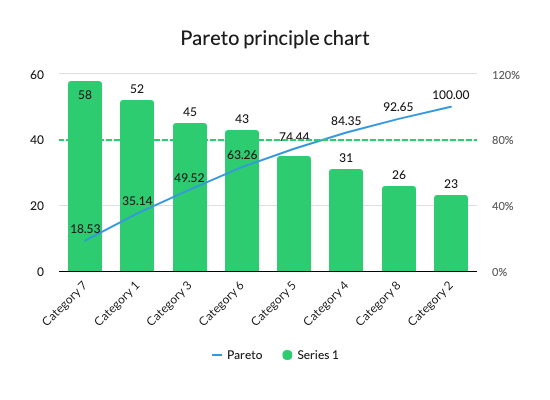THE PARETO PRINCIPLE

Finding the right focus and answer using the 80:20 rule

Pareto analysis emerged from Vilfredo Pareto’s observation that many activities break down to an 80:20 ratio, where 80% of production is due to 20% of contributing factors. This observation is now being used to focus business strategies, problem solving and operations on the key inputs that account for 80% of the outcome.

How it works

The 80:20 ratio is applicable to both positive and negative situations, offering a helpful way to deal with problems or opportunities rapidly. In other words, by identifying the few key factors that contribute greatly to a scenario, we can better focus efforts in order to obtain the required result Pareto analysis is only as good as the data used, so we need to ensure that all contributing factors are identified and that the parameters and measures that are suitable and revealed are properly defined and interpreted. Although not all falls neatly into a rule of 80:20, analyzing Pareto is still useful in identifying the main causal factors.

This simple instance demonstrates how it works.

•  Research and discuss all contributing factors.
• Decide suitable time period and measurement method.
• Measure how often each factor occurs (or another measure, like cost).
• Rank the factors in the descending order.
• Calculate frequency of each factor as a proportion of the total occurrences (or cost).
• Calculate the cumulative percentage (current percentage plus any previous percentage).Depict this information on a graph with ‘frequency as a percentage of total’ as a bar chart and ‘cumulative percentage’ as a line, adding a third line showing the 80 percent cut-off point.

Factors that appear to the left of the intersection of the two hues are the ones contributing to 80 percent of the result – these are the factors to focus on.

Example of how the Pareto Principle can be displayedA chart to display Pareto principle and evaluate which category/series meets the 80% criteria

October 2, 2019